Asymptotic behaviour of the Direct Interaction Approximation.
As mentioned previously, Kraichnan’s asymptotic solution of the DIA, for high Reynolds numbers and large wavenumbers, did not agree with the observed asymptotic behaviour of turbulence. His expression for the spectrum was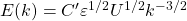, where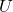is the root-mean-square velocity and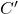is a constant. In 1964 (see  for the reference) he wrote: Recent experimental evidence gives strong support to [the Kolmogorov -5’3’ form] and rules out [the -3/2’ form above] as a correct asymptotic law.’

However, Kraichnan’s result is not actually an asymptotic form. The rms velocityis in fact part of the solution, not the initial conditions. We may underline this by writing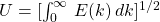, which allows us to rewrite the Kraichnan result as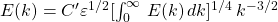. So, far from being an asymptotic solution, this appears to be a form of transcendental equation for the energy spectrum.

Now you may object that the dissipation rate is also part of the solution, rather than of the initial conditions, and hence this is also a criticism of the Kolmogorov form. But this is not so. The dissipation only appears because it is equal to the inertial transfer rate. From the simple physics of the inertial range in wavenumber space, the appropriate quantity is the maximum value of the inertial flux of energy through modes, which we will denote by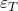. Hence the Kolmogorov form should really be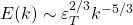. Of course Kolmogorov worked in real space and derived the 2/3’ law. But in 1941 Obukhov recognised that in wavenumber space the relevant quantity was the scale-invariant energy flux, as did Onsager a few years later.

A way of putting the Kraichnan result in a more asymptotic form was given by McComb and Yoffe , who made use of the asymptotic Taylor surrogate for the dissipation rate,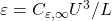, where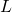is the integral length scale and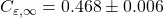, to substitute forin the Kraichnan spectrum, and obtained: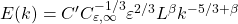, where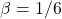. Note that we have changedin that reference to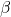in order to avoid any confusion with the so-called intermittency correction, which normally is represented by that symbol.

Kraichnan only computed the Eulerian DIA for free decay at low Reynolds numbers. However, in 1989 McComb, Shanmugasundaram and Hutchinson  reported calculations for free decay of both DIA and LET for Taylor-Reynolds numbers in the range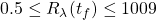where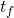is the final time of the computation. These results do not support the asymptotic form of the DIA energy spectrum, as given above. It was found that (for example) at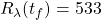, the two theories were virtually indistinguishable and both gave the Kolmogorov spectrum to within the accuracy of the numerical methods. It was shown that this result was not an artefact of the initial conditions, by taking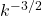as the initial spectrum, whereupon it was found that both theories evolved away from this form to once again give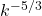as the final spectrum.

There is much that remains to be understood about Eulerian turbulence theories and the behaviour of two-time correlations.

 W. D. McComb and S. R. Yoffe. A formal derivation of the local energy transfer (LET) theory of homogeneous turbulence. J. Phys. A: Math. Theor., 50:375501, 2017.
 W. D. McComb, A. Berera, S. R. Yoffe, and M. F. Linkmann. Energy transfer and dissipation in forced isotropic turbulence. Phys. Rev. E, 91:043013, 2015.
 W. D. McComb, V. Shanmugasundaram, and P. Hutchinson. Velocity derivative skewness and two-time velocity correlations of isotropic turbulence as predicted by the LET theory. J. Fluid Mech., 208:91, 1989.